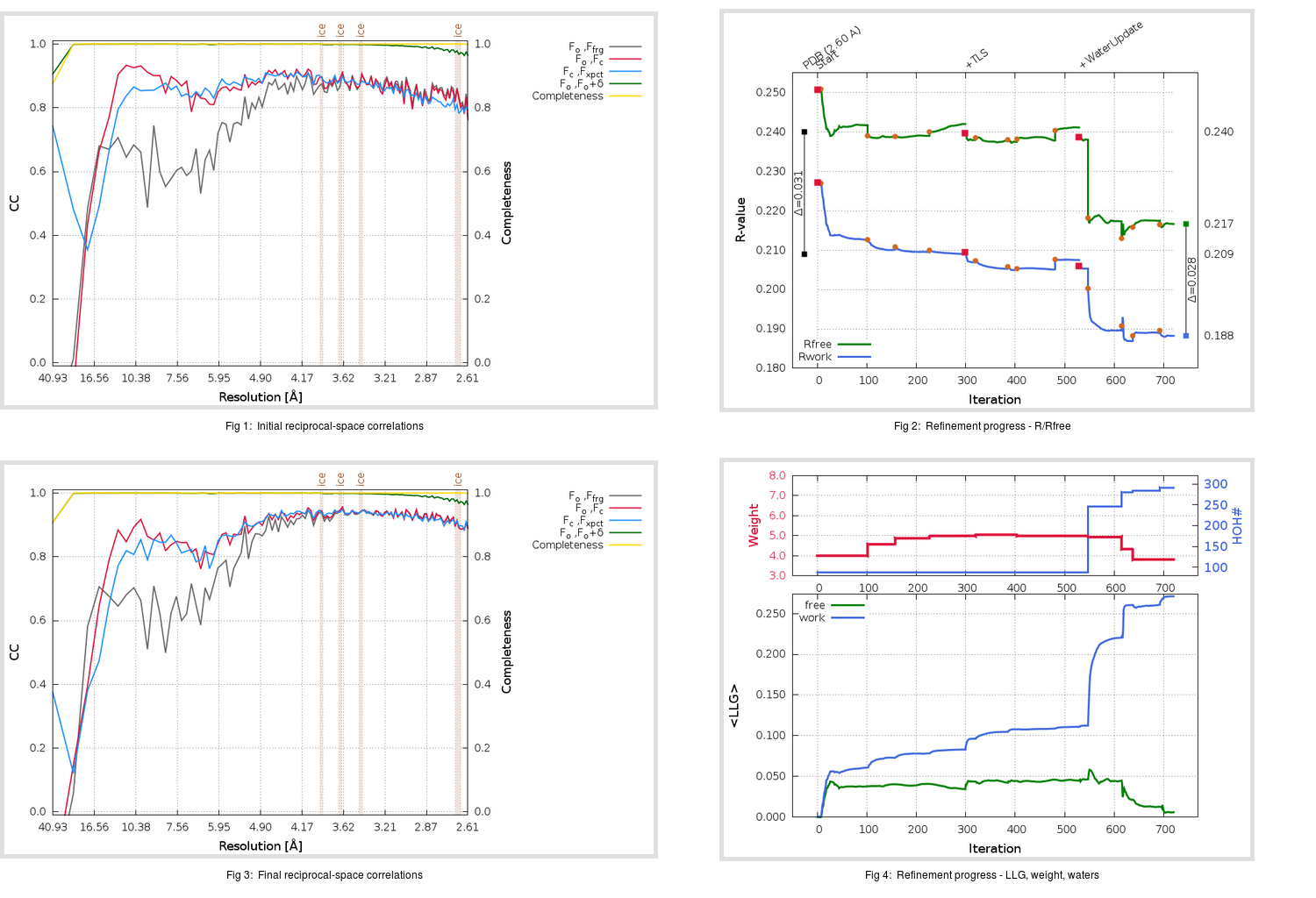Content:

```    Diffraction limits & principal axes of ellipsoid fitted to diffraction cut-off surface:
2.574         1.0000   0.0000   0.0000       a*
2.574         0.0000   1.0000   0.0000       b*
2.574         0.0000   0.0000   1.0000       c*
```

## Deposited

` `
 Date deposited Date data collection Resolution R, Rfree 20060606 20051103 2.60 0.2080 0.2400

Molprobity (CCP4 7.0 version) summary:

```Ramachandran outliers =   0.29 %
favored =  98.26 %
Rotamer outliers      =   9.93 %
C-beta deviations     =     1
Clashscore            =   4.98
RMS(bonds)            =   0.0102
RMS(angles)           =   1.30
MolProbity score      =   2.02
Resolution            =   2.60
R-work                =   0.2080
R-free                =   0.2400
```

```Number of waters      =    88

<B> (all atoms) =   30.42 ( sd =    6.71 ) for       2802 non-hydrogen atoms
<B>   (protein) =   30.55 ( sd =    6.66 ) for       2714 non-hydrogen atoms
<B>     (water) =   26.18 ( sd =    6.67 ) for         88 non-hydrogen atoms
<B>    (others) =    0.00 ( sd =    0.00 ) for          0 non-hydrogen atoms

B min/max       (all non-hydrogen atoms) =   11.40 /   51.54
B min/max   (protein non-hydrogen atoms) =   16.95 /   51.54
B min/max     (water non-hydrogen atoms) =   11.40 /   44.95
B min/max     (other non-hydrogen atoms) =    0.00 /    0.00
```

## BUSTER (re-)refinement

` `

Molprobity (CCP4 7.0 version) summary:

```Ramachandran outliers =   0.00 %
favored =  98.55 %
Rotamer outliers      =   9.27 %
C-beta deviations     =     5
Clashscore            =   5.17
RMS(bonds)            =   0.0134
RMS(angles)           =   1.66
MolProbity score      =   2.01
Resolution            =   2.60
R-work                =   0.1882
R-free                =   0.2166
```

```Number of waters      =   291

<B> (all atoms) =   34.82 ( sd =   11.01 ) for       3005 non-hydrogen atoms
<B>   (protein) =   33.51 ( sd =    9.76 ) for       2714 non-hydrogen atoms
<B>     (water) =   47.03 ( sd =   14.15 ) for        291 non-hydrogen atoms
<B>    (others) =    0.00 ( sd =    0.00 ) for          0 non-hydrogen atoms

B min/max       (all non-hydrogen atoms) =   15.28 /   94.04
B min/max   (protein non-hydrogen atoms) =   15.51 /   74.08
B min/max     (water non-hydrogen atoms) =   15.28 /   94.04
B min/max     (other non-hydrogen atoms) =    0.00 /    0.00
```

Refinement progression:Results:

` `
 File Remark 2H85_aB_refine.01_03_refine.pdb.gz exact refinement commands are in header 2H85_aB_refine.01_03_refine.mtz.gz including original deposited data and several re-refinement map coefficients 2H85_aB_refine.01_03_BUSTER_model.cif.gz including any non-standard compound restraints 2H85_aB_refine.01_03_BUSTER_refln.cif.gz